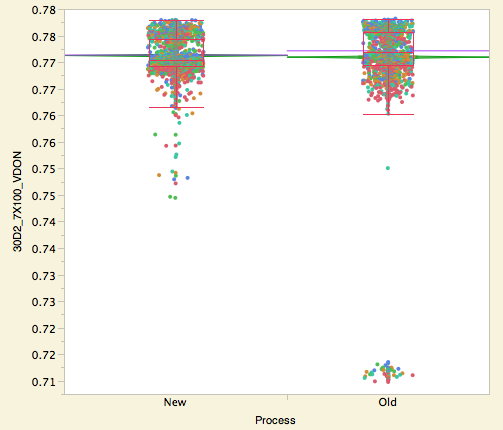Our World Statistics Day conversations have been a great reminder of how much statistics can inform our lives. Do you have an example of how statistics has made a difference in your life? Share your story with the Community!
Choose Language Hide Translation Bar
Bad data happens to good people: Robust to outliers

In semiconductor data, it is common for probe measurements that encounter an electrical short to exhibit measurements that are far out in the distribution, i.e., they are outliers. When we test that means are the same, these outlying values inflate our estimate of the standard deviation [sigma]. Remember that the standard deviation is estimated using the squared distances of each point from its predicted value, and thus far-outlying values have a disproportionately large effect on the standard deviation estimate. Because of the inflated variance estimate, they also usually make tests of means less sensitive, resulting in larger less-significant p-values.

Fortunately, there are methods to compensate for these outliers: robust methods. The robust method we like is the Huber M Estimator. Basically, this estimates means and a sigma such that when points are farther than two sigma from the mean, they are down-weighted so that the calculations are sensitive as the absolute value, rather than the square of the deviation.

Robust methods are more expensive to calculate. The Huber-M estimator is an iterative method that cycles through the data many times to obtain estimates and tests. But we have fast computers now, so there is no excuse in most cases for not considering robust estimates.

I did a robust fit on the lot-to-lot variation on the production data, all 118 variables. On the left is the relationship of the robust LogWorth by the non-robust LogWorth. The 45-degree line is where they are equal. They are pretty equal, with all the points near the line. The data seems well-behaved, not infected by outliers.

But in the engineering-change data, all 384 responses, in the corresponding plot on the right, the LogWorths are very significant, and most of them are very different when estimated robustly. Most of the tests are far more significant when done robustly.

Let’s pick one of the tests where the robust test is significant, but the non-robust is not.The non-robust ANOVA p-value is .1007, and the robust ANOVA p-value is 5.98E-16 – much different. Not only that, but when fit non-robustly, the mean for “new” is larger. But for the robust version, down-weighting all those outliers in the Old group, the mean for “old” is larger, the opposite order. (The non-robust means are green lines, the robust ones lavender). It turns out that tests don’t always get more significant when estimated robustly; in the 387 tests in this example, 138 of them have robust tests less significant that non-robust.

The lesson is that unless we know that the data is well-behaved, we should try using robust estimates. Otherwise, we risk being blinded to real differences that standard (non-robust) methods don’t detect.

JMP 11 has robust Huber M methods in many new places: Distribution, Oneway, Bivariate, Response Screening and Fit Model - Response Screening personality.

Note: This is part of a Big Statistics series of blog posts by John Sall. Read all of his Big Statistics posts.

Article Labels

There are no labels assigned to this post.

Article Tags